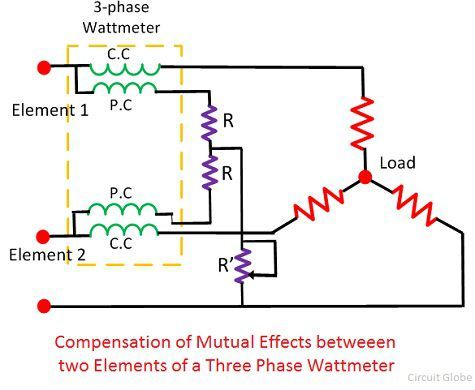# Three Phase Wattmeter

Definition: Three-Phase Wattmeter is used for measuring the power of the three-phase circuit. In three-phase Wattmeter, the two separate Wattmeter are mounted together in the single unit. Their moving coils are placed on the same spindle.

Three-Phase Wattmeter has two elements. The single element is the combination of the pressure coil and the current coil. The current coils are considered as the fixed coil, and the pressure coils are the moving coil of the Wattmeter.

## Working Principle of Three Phase Wattmeter

It works on the principle that the torque develops on the current carrying conductor when it is placed in the magnetic field. The measurand power when passes through the moving coils, the torque develops on the coil. The torque is the type of mechanical force whose effect can deflect the object in circular motion.

In three-phase Wattmeter, the torque develops on both the elements. The value of torque on each element is proportional to the power passes through it. The total torque on the three-phase Wattmeter is the sum of the torque on individual Wattmeter.

Let understand this with the help of the mathematical expressions.

Consider the deflecting torque develops on the coil one is D1 and the power passes through that element is P1. Similarly, the torque develops on the coil 2 is D2 and the power passes through the coil is P2.The total torque develops in the coil is expressed as

###Connections of three-phase Wattmeter

Consider the circuit has two Wattmeters. The current coil of both the Wattmeter connects across any two phases say R and Y. The pressure coil of both the Wattmeters connects across the third phase say B.

The mutual interference between the elements of the Three-Phase Wattmeter will affect their accuracy. The mutual interference is the phenomena in which the field of two elements interacts each other. In three-phase Wattmeter, the laminated iron shield is placed between the elements. The iron shield reduces the mutual effect of the element.The mutual effect can compensate by using the Weston method. In Weston method, the adjustable resistors are used. This resistor compensates the mutual interference that occurs between the elements of three phase wattmeter.

### 4 thoughts on “Three Phase Wattmeter”

1. Ghazanfar Ali Khan

I have studied three articles on this circuit globe and found very useful. Thank for that and keep me in the list for future electrical system studies.

2. articles are very useful for understanding as for students. It will be more beneficial for us to understood the concepts related to electrical engineering.